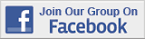Excel Tips & Tutorials:
Logistic Regression
 Suggest a Link Alphabetize Page Printer-Friendly List
Watch and Learn
Watch and Learn
Watch and Learn
Watch and Learn
 Logistic Regression Using ExcelAnalyzes who survived Titanic disaster using Real Statistics Add-In Logistic Regression Tool (7:54).ROC Curves and AUC ExplainedHow to visualize the performance of a binary classifier, useful with logistic regression (14:05).Binary Logistic RegressionUses Real Statistics Add-In to analyze business example (4:48).Logistic Regression Analysis Using Optimization with SolverVikas Agrawal discusses concept of logistic regression and implements with Solver (16:26).Logistic Regression Using ExcelAnalyzes who survived Titanic disaster using Real Statistics Add-In Logistic Regression Tool (7:54).ROC Curves and AUC ExplainedHow to visualize the performance of a binary classifier, useful with logistic regression (14:05).Binary Logistic RegressionUses Real Statistics Add-In to analyze business example (4:48).Logistic Regression Using ExcelAnalyzes who survived Titanic disaster using Real Statistics Add-In Logistic Regression Tool (7:54).ROC Curves and AUC ExplainedHow to visualize the performance of a binary classifier, useful with logistic regression (14:05).Logistic Regression Using ExcelAnalyzes who survived Titanic disaster using Real Statistics Add-In Logistic Regression Tool (7:54).Logistic Regression Reference Detailed reference from Real Statistics Using Excel, covers logistic regression Add-In.Logistic Regression Reference Detailed reference from Real Statistics Using Excel, covers logistic regression Add-In.Logistic Regression Reference Detailed reference from Real Statistics Using Excel, covers logistic regression Add-In.Logistic Regression Reference Detailed reference from Real Statistics Using Excel, covers logistic regression Add-In.Over 18,000 Members!